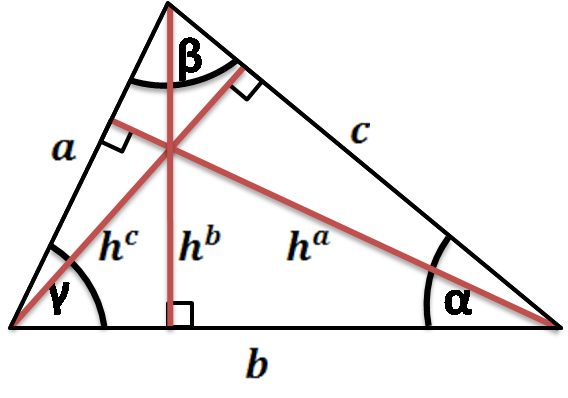# Scalene Triangle Calculator

Created by Łucja Zaborowska, MD, PhD candidate
Last updated: Jun 03, 2022

Looking for the ultimate scalene triangle calculator? You've definitely come to the right place!

The next 300 words will introduce you to the world of scalene triangle solver and every possible irregular triangle area calculation - keep on reading to discover:

This scalene triangle solver is based on a similar triangle height calculator. 💎

## What is a scalene triangle?

A scalene triangle is a triangle that comprises three unequal sides. To put it simply, all of its sides have completely different lengths.

The scalene triangle calculator on your left allows you to calculate the area of a scalene triangle, as well as its perimeter, sides, heights and angles!

## How do I calculate the perimeter of a scalene triangle?

Calculating the perimeter of a scalene triangle is extremely simple!

Perimeter = a + b + c

Where:

• a, b, and c are sides of the scalene triangle.
1. Enjoy the results - you've finished the easiest part of scalene triangle calculations! 🎉

## How to calculate the area of a scalene triangle?

We may use multiple equations - the area of a scalene triangle is calculated in exactly the same way as for all the triangles.

Let's choose the equation that suits you best:

• Area = 0.5 × Base × Height

• Area = 0.5 × a × b × sin(γ)

• Area = 0.25 × √((a + b + c) × (-a + b + c) × (a - b + c) × (a + b - c))

• Area = a² × sin(β) × sin(γ)/ (2 × sin(β + γ))

## How to calculate a scalene triangle's height?

There are a few scalene triangle equations that may be of help:

• Calculate height for any triangle:

Height = 2 × Area/ Base

Height = 0.5 × √((a + b + c) × (-a + b + c) × (a - b + c) × (a + b - c)) / b

• For the right triangle:

Heightᶜ = a × b / c

Where:

• a, b, and c are sides of the triangle; and
• Heightᶜ is the height of a base c.

## How to calculate the area of a scalene right triangle?

The formula for the area of a scalene right triangle is just the same as for any other right triangle:

Area = a × b / 2

or

Area = b × √(c² - b²) / 2

Where:

• a and b are the perpendicular sides; and
• c is the hypotenuse.
Łucja Zaborowska, MD, PhD candidate
Special triangle?
noSides
a
in
b
in
c
in
Heights (altitudes)
hᵃ
in
hᵇ
in
hᶜ
in
Angles
α
deg
β
deg
γ
deg
Others
Area
in²
Perimeter
in
People also viewed…

### Books vs e-Books